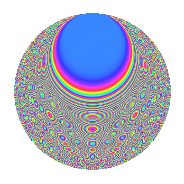# Properties

 Label 450.2.vLevel 450 Weight 2 Character orbit v Rep. character $$\chi_{450}(79,\cdot)$$ Character field $$\Q(\zeta_{30})$$ Dimension 240 Newform subspaces 1 Sturm bound 180 Trace bound 0

# Related objects

## Defining parameters

 Level: $$N$$ = $$450 = 2 \cdot 3^{2} \cdot 5^{2}$$ Weight: $$k$$ = $$2$$ Character orbit: $$[\chi]$$ = 450.v (of order $$30$$ and degree $$8$$) Character conductor: $$\operatorname{cond}(\chi)$$ = $$225$$ Character field: $$\Q(\zeta_{30})$$ Newform subspaces: $$1$$ Sturm bound: $$180$$ Trace bound: $$0$$

## Dimensions

The following table gives the dimensions of various subspaces of $$M_{2}(450, [\chi])$$.

Total New Old
Modular forms 752 240 512
Cusp forms 688 240 448
Eisenstein series 64 0 64

## Trace form

 $$240q - 30q^{4} - 8q^{5} + 4q^{9} + O(q^{10})$$ $$240q - 30q^{4} - 8q^{5} + 4q^{9} - 4q^{11} + 10q^{12} + 8q^{14} - 20q^{15} + 30q^{16} - 2q^{20} + 24q^{21} + 24q^{25} - 96q^{26} + 30q^{27} + 12q^{29} - 22q^{30} + 12q^{31} + 50q^{33} - 32q^{35} + 8q^{36} - 52q^{39} - 16q^{41} - 8q^{44} - 108q^{45} - 50q^{47} - 20q^{48} + 120q^{49} - 4q^{50} - 32q^{51} - 24q^{54} + 24q^{55} - 8q^{56} + 18q^{59} + 6q^{60} - 60q^{62} - 70q^{63} + 60q^{64} - 64q^{65} - 16q^{66} - 30q^{67} - 8q^{69} + 24q^{70} + 76q^{71} - 80q^{74} - 6q^{75} + 80q^{77} - 20q^{78} + 12q^{79} - 4q^{80} - 36q^{81} - 140q^{83} - 18q^{84} + 12q^{85} - 20q^{86} - 150q^{87} - 28q^{89} + 62q^{90} - 40q^{92} + 36q^{95} - 28q^{99} + O(q^{100})$$

## Decomposition of $$S_{2}^{\mathrm{new}}(450, [\chi])$$ into newform subspaces

Label Dim. $$A$$ Field CM Traces $q$-expansion
$$a_2$$ $$a_3$$ $$a_5$$ $$a_7$$
450.2.v.a $$240$$ $$3.593$$ None $$0$$ $$0$$ $$-8$$ $$0$$

## Decomposition of $$S_{2}^{\mathrm{old}}(450, [\chi])$$ into lower level spaces

$$S_{2}^{\mathrm{old}}(450, [\chi]) \cong$$ $$S_{2}^{\mathrm{new}}(225, [\chi])$$$$^{\oplus 2}$$

## Hecke Characteristic Polynomials

There are no characteristic polynomials of Hecke operators in the database